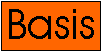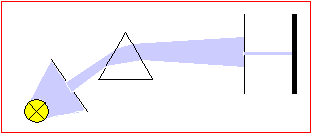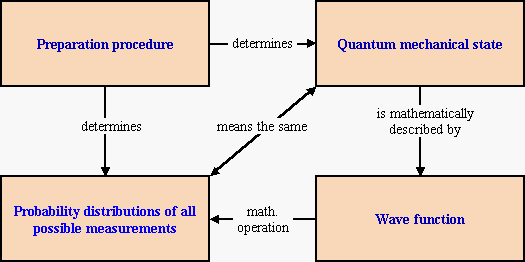##Preparation of quantum mechanical states

Preparation means the production of certain initial conditions on a physical object. An example is the production of light of a certain color in Newton`s prism experiment:The light that is prepared in this experiment possesses a certain dynamical property (color or wavelength). By preparation, physical objects with definite dynamical properties are produced. When a system has a certain property this property will show up in a test measurement (in the prism experiment, this can be performed by a second prism). Only if it is possible to predict with certainty that an object will show a certain property in a test is one  allowed to ascribe this property to the physical object itself. In this case (and only in this case) does the object really possess this property.

Complete preparation leads to reproducible predictions about the probabilities of all possible measurements on the physical object. To make this connection between preparation and measured probability distributions more explicit, one introduces the notion of the quantum mechanical state. The state is identified with the specification of a probability distribution for each possible measurement. Mathematically, a quantum mechanical state is described by a wave function. With certain mathematical operations, it is possible to extract from the wave fuction the probabilities of all conceivable experiments. The conceptual connection between preparation, state, wave function and probability distribution is illustrated in the graphics below.To get back to the current lesson use the back button of your browser.